Share

# Reflection of Light - Spherical Mirrors

Course

#### description

Brief introduction to spherical mirrors - concave and convex mirrors, centre and radius of curvature, pole and principal axis, focus and focal length

#### notes

Types of Mirror:

Plain Mirror: A mirror having a flat surface is called plane mirror.

Formation of image in plane mirror: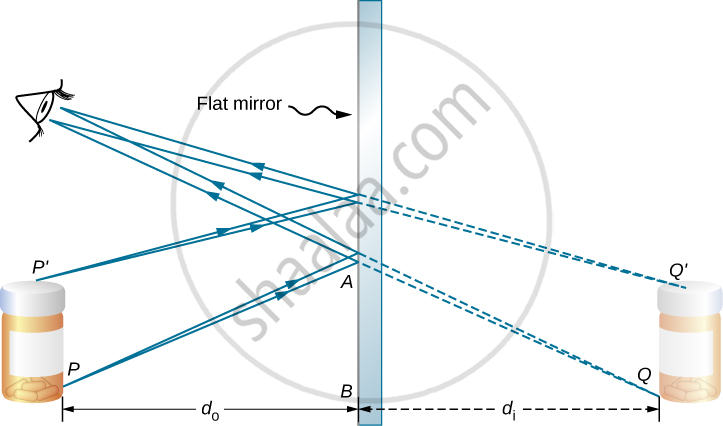• A plane mirror always forms virtual and erect image.

• The distance of image and that of object is equal from the mirror.

• The image formed by a plane mirror is laterally inverted

Spherical Mirror: Mirrors having curved reflecting surface are called spherical mirrors. A spherical mirror is a part of a sphere.

#### Types of Spherical Mirror:

Concave Mirror: Spherical mirror with reflecting surface curved inwards is called concave mirror.

Convex Mirror: Spherical mirror with reflecting surface curved outwards is called convex mirror.

#### Important terms in the case of spherical mirror: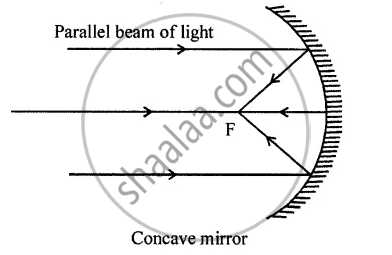Pole: The centre of reflecting surface of a spherical mirror is known as Pole. Pole lies on the surface of spherical mirror. Pole is generally represented by ‘P’.

Centre of Curvature: The centre of sphere; of which the reflecting surface of a spherical mirror is a part; is called the centre of curvature of the spherical mirror. Centre of curvature is not a part of spherical mirror rather it lies outside the mirror. Centre of curvature is denoted by letter ‘C’.

In the case of concave mirror centre of curvature lies in front of the reflecting surface. On the other hand, centre of curvature lies behind the reflecting surface in the case of convex mirror.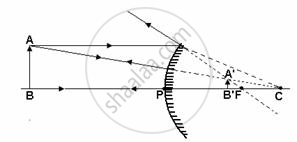Radius of Curvature: The radius of sphere; of which the reflecting surface of a spherical mirror is a part; is called the Radius of Curvature of the spherical mirror. The radius of curvature of a spherical mirror is denoted by letter ‘R’.

Similar to centre of curvature, radius of curvature lies in front of concave mirror and lies behind the convex mirror and is not a part of the mirror as it lies outside the mirror.

Aperture: The diameter of reflecting surface of a spherical mirror is called aperture.

Focus or Principal Focus: Point on principal axis at which parallel rays; coming from infinity; converge after reflection is called the Focus or Principal Focus of the spherical mirror. Focus is represented by letter ‘F’.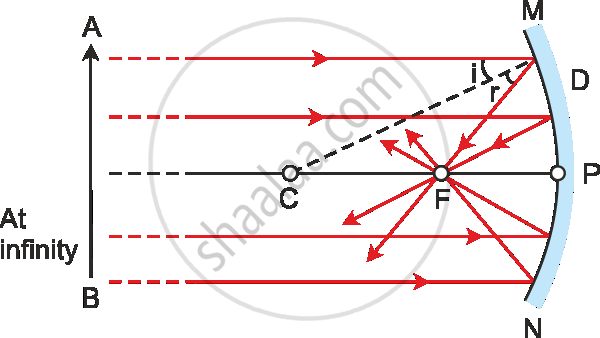Principal Axis: Imaginary line passing through the centre of curvature and pole of a spherical mirror is called the Principal Axis.

In the case of a concave mirror, parallel rays; coming from infinity; converge after reflection in front of the mirror. Thus, the focus lies in front of a concave mirror.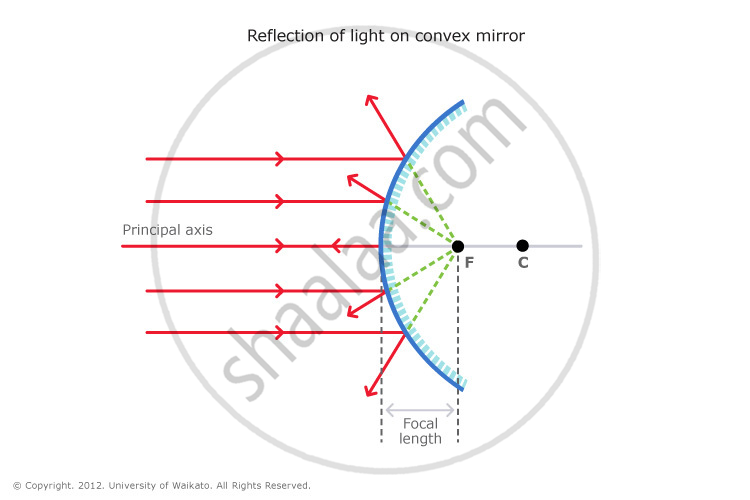In the case of a convex mirror, parallel rays; coming from infinity; appear to be diverging from behind the mirror. Thus, the focus lies behind the convex mirror.

Focal length: The distance from pole to focus is called focal length. Focal length is denoted by letter ‘f’. Focal length is equal to half of the radius of curvature.

Or, f=R/2

Or, R=2f

### Shaalaa.com

Light Reflection and Refraction part 3 (Spherical Mirror) [00:12:08]
S
series 1
0%

S